Max / Min
Roots & Intercepts
Domain & Range
AOS & Vertex
Random
100Minimum

100

What are the roots of the graph?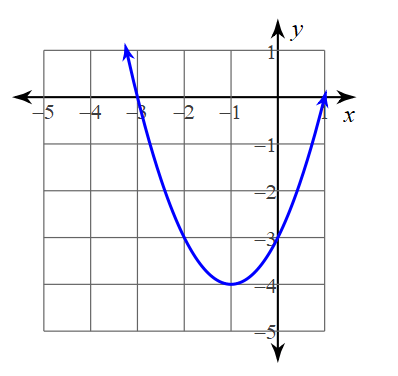(-3, 0) and ( 1, 0)

100

What the domain of the graph?All Real numbers

100

What is the Axis of symmetry?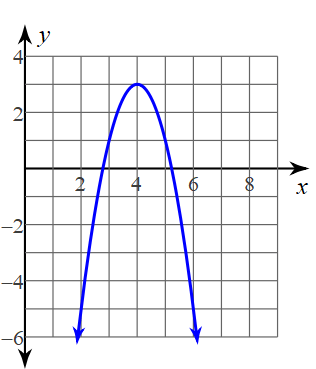x = 4

100

What are the factors of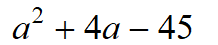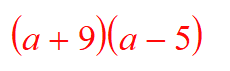200Maximum

200

What are the zeros of the graph?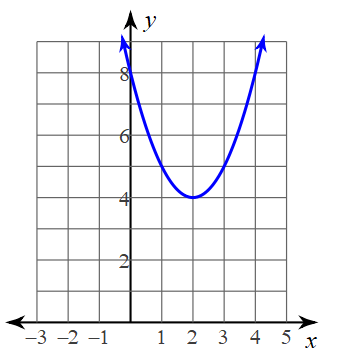No zeros

200

What is the range?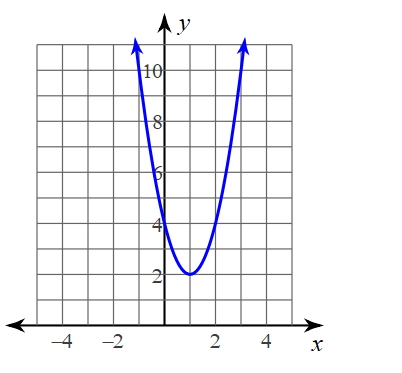Numbers greater than or equal to 2

200

What is the vertex?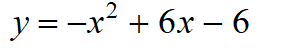(3,3)

200

How many children does your teacher have?

3

300Minimum

300

What are the solutions of the graph?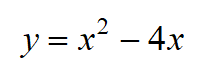(0, 0) and (4, 0)

300

What is the domain and range?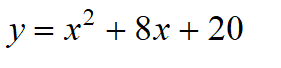Domain: All real numbers

Range: y ≥ 4

300

What is the AOS and Vertex ?Vertex : (1, -5)

AOS: x = 1

300

What is the domain for all parabolas?

All real numbers

400

A U shaped parabola.

Minimum

400

What is the y intercept ?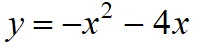( 0, 0)

400

What is the domain and range?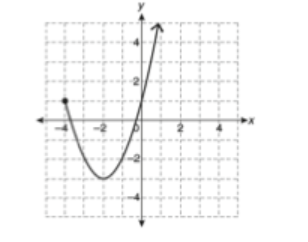Domain : x ≥ - 4

Range : y ≥  -3

400

What is the AOS and vertex of ?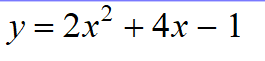Vertex:  (-1, -3)

AOS: x = -1

400

What is f(3)?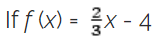-2

500

A parabola that opens down.

Maximum

500

What is the y intercept?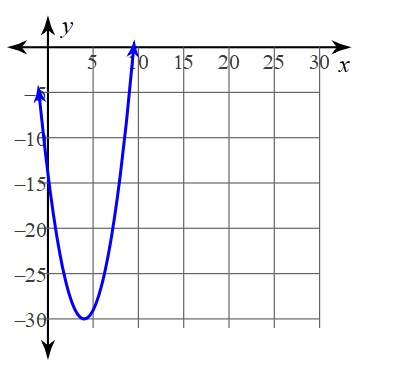(0, -15)

500

What is the domain and range?Domain: -5 < x  ≤ 1

Range: -3 ≤ y ≤ 1

500

What is the AOS and Vertex?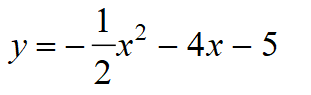Vertex: (-4, 3)

AOS: x= -4

500

What is the slope of the table.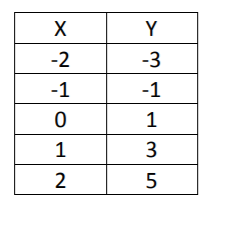slope is : 2

Click to zoom